### Example 59.9 Examining Individual Test Components

The LCOMPONENTS option in the MODEL statement enables you to perform single-degree-of-freedom tests for individual rows of thematrix. Such tests are useful to identify interaction patterns. In a balanced layout, Type 3 components ofassociated with A*B interactions correspond to simple contrasts of cell mean differences.

The first example revisits the data from the split-plot design by Stroup (1989a) that was analyzed in Example 59.1. Recall that variables A and B in the following statements represent the whole-plot and subplot factors, respectively:

proc mixed data=sp;
class a b block;
model y = a b a*b / LComponents e3;
random block a*block;
run;


The MIXED procedure constructs a separatematrix for each of the three fixed-effects components. The matrices are displayed in Output 59.9.1. The tests for fixed effects are shown in Output 59.9.2.

Output 59.9.1: Coefficients of Type 3 Estimable Functions

The Mixed Procedure

Type 3 Coefficients for A
Effect A B Row1 Row2
Intercept
A 1   1
A 2     1
A 3   -1 -1
B   1
B   2
A*B 1 1 0.5
A*B 1 2 0.5
A*B 2 1   0.5
A*B 2 2   0.5
A*B 3 1 -0.5 -0.5
A*B 3 2 -0.5 -0.5

Type 3 Coefficients for B
Effect A B Row1
Intercept
A 1
A 2
A 3
B   1 1
B   2 -1
A*B 1 1 0.3333
A*B 1 2 -0.333
A*B 2 1 0.3333
A*B 2 2 -0.333
A*B 3 1 0.3333
A*B 3 2 -0.333

Type 3 Coefficients for A*B
Effect A B Row1 Row2
Intercept
A 1
A 2
A 3
B   1
B   2
A*B 1 1 1
A*B 1 2 -1
A*B 2 1   1
A*B 2 2   -1
A*B 3 1 -1 -1
A*B 3 2 1 1

Output 59.9.2: Type 3 Tests in Split-Plot Example

Type 3 Tests of Fixed Effects
Effect Num DF Den DF F Value Pr > F
A 2 6 4.07 0.0764
B 1 9 19.39 0.0017
A*B 2 9 4.02 0.0566

If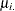denotes a whole-plot main effect mean,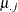denotes a subplot main effect mean, and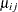denotes a cell mean, the five components shown in Output 59.9.3 correspond to tests of the following:

•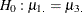•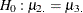•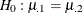•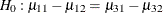•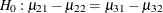Output 59.9.3: Type 3 L Components Table

L Components of Type 3 Tests of Fixed Effects
Effect L Index Estimate Standard Error DF t Value Pr > |t|
A 1 7.1250 3.1672 6 2.25 0.0655
A 2 8.3750 3.1672 6 2.64 0.0383
B 1 5.5000 1.2491 9 4.40 0.0017
A*B 1 7.7500 3.0596 9 2.53 0.0321
A*B 2 7.2500 3.0596 9 2.37 0.0419

The first three components are comparisons of marginal means. The fourth component compares the effect of factor B at the first whole-plot level against the effect of B at the third whole-plot level. Finally, the last component tests whether the factor B effect changes between the second and third whole-plot level.

The Type 3 component tests can also be produced with these corresponding ESTIMATE statements:

proc mixed data=sp;
class a b block ;
model y = a b a*b;
random block a*block;
estimate 'a    1' a 1 0 -1;
estimate 'a    2' a 0 1 -1;
estimate 'b    1' b   1 -1;
estimate 'a*b  1' a*b 1 -1 0  0 -1 1;
estimate 'a*b  2' a*b 0  0 1 -1 -1 1;
ods select Estimates;
run;


The results are shown in Output 59.9.4.

Output 59.9.4: Results from ESTIMATE Statements

The Mixed Procedure

Estimates
Label Estimate Standard Error DF t Value Pr > |t|
a 1 7.1250 3.1672 6 2.25 0.0655
a 2 8.3750 3.1672 6 2.64 0.0383
b 1 5.5000 1.2491 9 4.40 0.0017
a*b 1 7.7500 3.0596 9 2.53 0.0321
a*b 2 7.2500 3.0596 9 2.37 0.0419

A second useful application of the LCOMPONENTS option is in polynomial models, where Type 1 tests are often used to test the entry of model terms sequentially. The SOLUTION option in the MODEL statement displays the regression coefficients that correspond to a Type 3 analysis. That is, the coefficients represent the partial coefficients you would get by adding the regressor variable last in a model containing all other effects, and the tests are identical to those in the Type 3 Tests of Fixed Effects table.

Consider the following DATA step and the fit of a third-order polynomial regression model.

data polynomial;
do x=1 to 20; input y@@; output; end;
datalines;
1.092   1.758   1.997   3.154   3.880
3.810   4.921   4.573   6.029   6.032
6.291   7.151   7.154   6.469   7.137
6.374   5.860   4.866   4.155   2.711
;


proc mixed data=polynomial;
model y = x x*x x*x*x / s lcomponents htype=1,3;
run;


The t tests displayed in the Solution for Fixed Effects table are Type 3 tests, sometimes referred to as partial tests. They measure the contribution of a regressor in the presence of all other regressor variables in the model.

Output 59.9.5: Parameter Estimates in Polynomial Model

The Mixed Procedure

Solution for Fixed Effects
Effect Estimate Standard Error DF t Value Pr > |t|
Intercept 0.7837 0.3545 16 2.21 0.0420
x 0.3726 0.1426 16 2.61 0.0189
x*x 0.04756 0.01558 16 3.05 0.0076
x*x*x -0.00306 0.000489 16 -6.27 <.0001

The Type 3 L components are identical to the tests in the Solutions for Fixed Effects table shown in Output 59.9.5. The Type 1 table yields the following:

• sequential (Type 1) tests of regression variables that test the significance of a regressor given all other variables preceding it in the model list

• the regression coefficients for sequential submodels

Output 59.9.6: Type 1 and Type 3 L Components

L Components of Type 1 Tests of Fixed Effects
Effect L Index Estimate Standard Error DF t Value Pr > |t|
x 1 0.1763 0.01259 16 14.01 <.0001
x*x 1 -0.04886 0.002449 16 -19.95 <.0001
x*x*x 1 -0.00306 0.000489 16 -6.27 <.0001

L Components of Type 3 Tests of Fixed Effects
Effect L Index Estimate Standard Error DF t Value Pr > |t|
x 1 0.3726 0.1426 16 2.61 0.0189
x*x 1 0.04756 0.01558 16 3.05 0.0076
x*x*x 1 -0.00306 0.000489 16 -6.27 <.0001

The estimate of 0.1763 is the regression coefficient in a simple linear regression of Y on X. The estimate of –0.04886 is the partial coefficient for the quadratic term when it is added to a model containing only a linear component. Similarly, the value –0.00306 is the partial coefficient for the cubic term when it is added to a model containing a linear and quadratic component. The last Type 1 component is always identical to the corresponding Type 3 component.